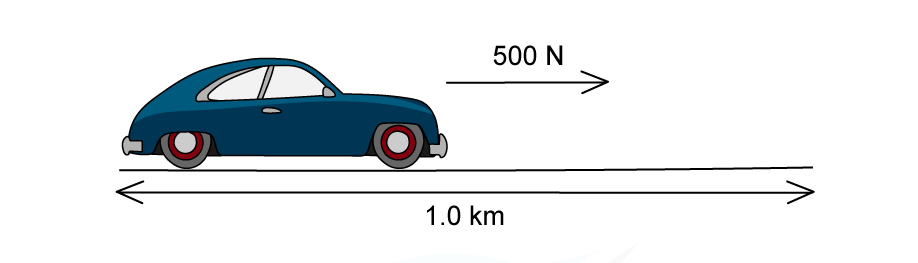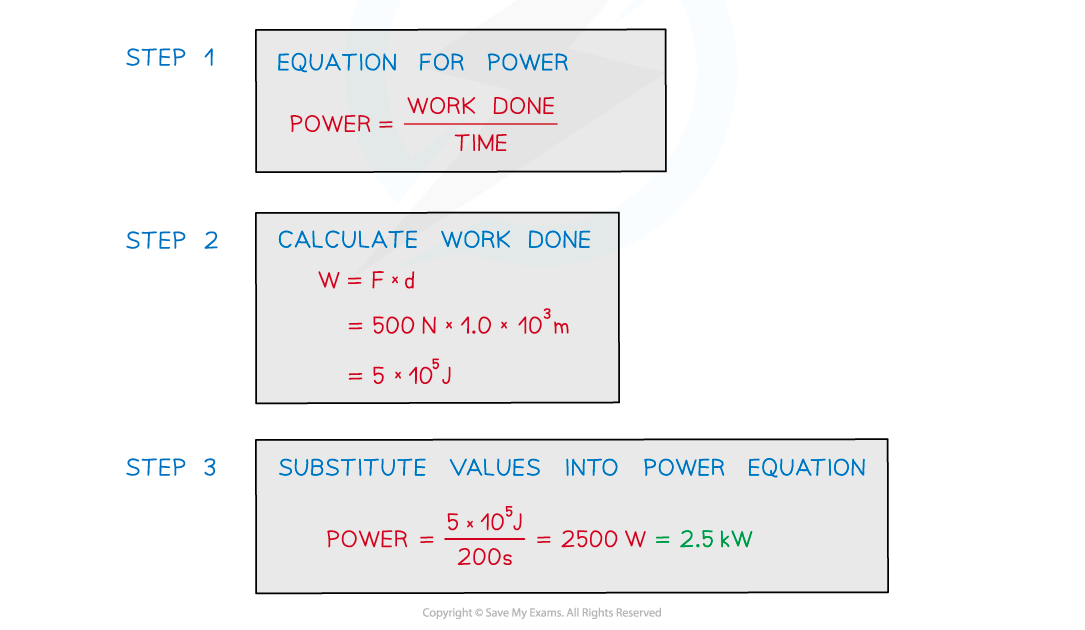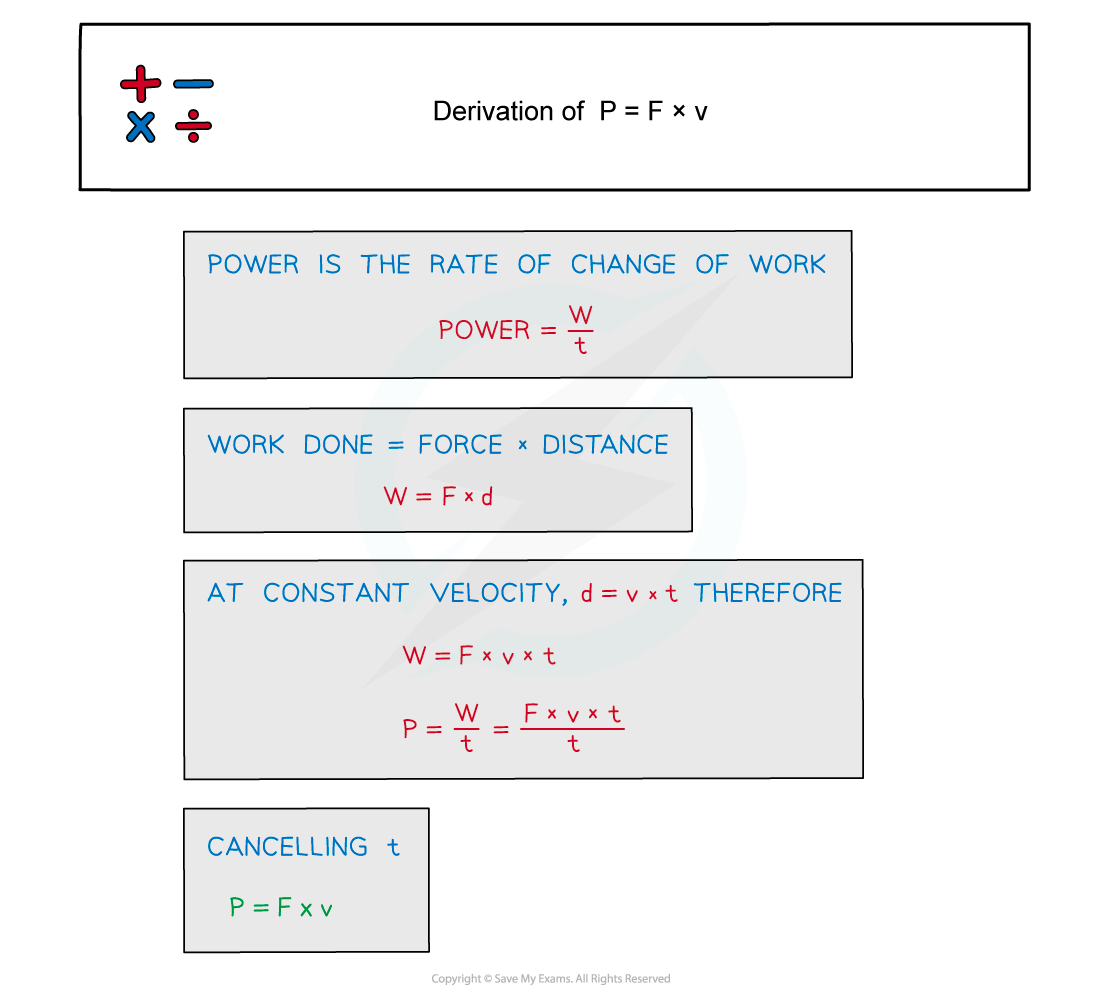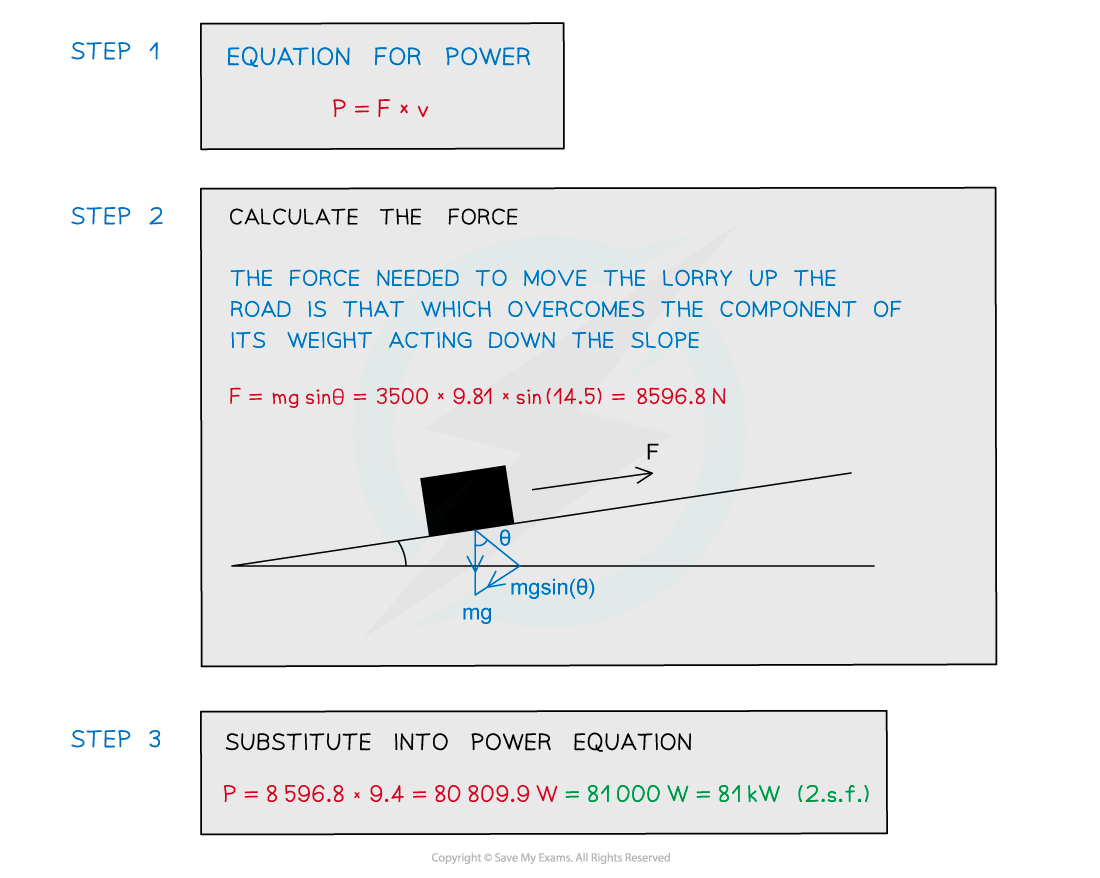# IB DP Physics: SL复习笔记2.3.5 Power

### Power

• The power of a mechanical process is the rate at which energy is transferred
• Since work done is equal to the energy transferred, power can also be defined as the rate of doing work or the work done per unit time
• Power can be calculated using the equation:• Power is also used in electricity, with labels on lightbulbs which indicate their power, such as 60 W or 100 W
• These indicate the amount of energy transferred by an electrical current rather than by a force doing work

#### The Watt

• Power is measured in Watts (W)
• The Watt, W, is commonly used as the unit power (and radiant flux)
• It is defined as 1 W = 1 J s–1
• The SI unit for energy is kg m2 s–3
• One watt is defined as:

A transfer of energy of 1 J in 1 s

#### Worked Example

A car engine exerts the following force for 1.0 km in 200 s.Determine what is the average power developed by the engine.#### Power & Force

• Moving power is defined by the equation:• This equation is only relevant where a constant force moves a body at constant velocity. Power is required in order to produce an acceleration
• The force must be applied in the same direction as the velocity

#### Derivation of P = Fv

• The derivation for this equation is shown below:#### Worked Example

A lorry moves up a road that is inclined at 14.5° to the horizontal.The lorry has a mass of 3500 kg and is travelling at a constant speed of 9.4 m s–1. The force due to air resistance is negligible.Calculate the useful power from the engine to move the lorry up the road.#### Exam Tip

The force represented in exam questions will often be a drag force. Whilst this is in the opposite direction to its velocity, remember the force needed to calculate the power is equal to (or above) this drag force to overcome it therefore you equate it to that value.